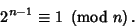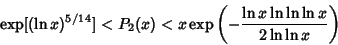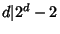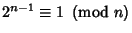## Poulet Number

A Fermat Pseudoprime to base 2, denoted psp(2), i.e., a Composite Odd Integer such thatThe first few Poulet numbers are 341, 561, 645, 1105, 1387, ... (Sloane's A001567). Pomerance et al. (1980) computed all 21,853 Poulet numbers less than.

Pomerance has shown that the number of Poulet numbers less thanfor sufficiently largesatisfy(Guy 1994).

A Poulet number all of whose Divisorssatisfyis called a Super-Poulet Number. There are an infinite number of Poulet numbers which are not Super-Poulet Numbers. Shanks (1993) calls any integer satisfying(i.e., not limited to Odd composite numbers) a Fermatian.

References

Guy, R. K. Unsolved Problems in Number Theory, 2nd ed. New York: Springer-Verlag, pp. 28-29, 1994.

Pomerance, C.; Selfridge, J. L.; and Wagstaff, S. S. Jr. The Pseudoprimes to.'' Math. Comput. 35, 1003-1026, 1980. Available electronically from ftp://sable.ox.ac.uk/pub/math/primes/ps2.Z.

Shanks, D. Solved and Unsolved Problems in Number Theory, 4th ed. New York: Chelsea, pp. 115-117, 1993.

Sloane, N. J. A. Sequence A001567/M5441 in An On-Line Version of the Encyclopedia of Integer Sequences.'' http://www.research.att.com/~njas/sequences/eisonline.html and Sloane, N. J. A. and Plouffe, S. The Encyclopedia of Integer Sequences. San Diego: Academic Press, 1995.